# StateSpace Search Artificial Intelligence Programming in Prolog Lecturer

• Slides: 22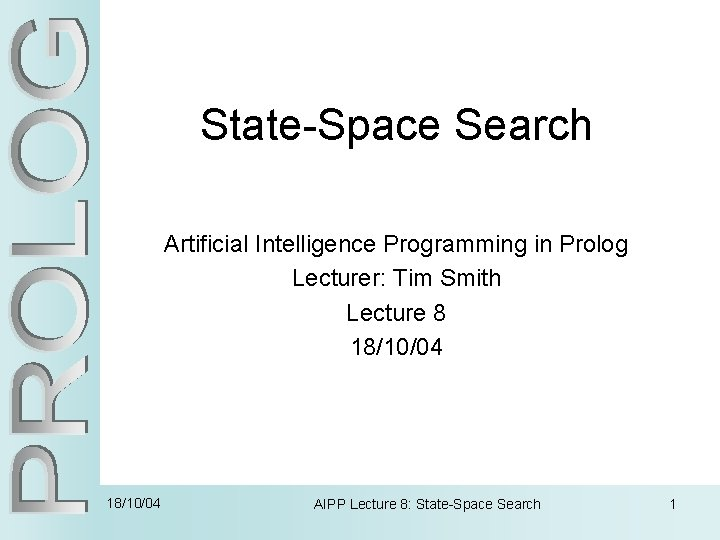State-Space Search Artificial Intelligence Programming in Prolog Lecturer: Tim Smith Lecture 8 18/10/04 AIPP Lecture 8: State-Space Search 1State-Space Search • Many problems in AI take the form of state-space search. • The states might be legal board configurations in a game, towns and cities in some sort of route map, collections of mathematical propositions, etc. • The state-space is the configuration of the possible states and how they connect to each other e. g. the legal moves between states. • When we don't have an algorithm which tells us definitively how to negotiate the state-space we need to search the statespace to find an optimal path from a start state to a goal state. • We can only decide what to do (or where to go), by considering the possible moves from the current state, and trying to look ahead as far as possible. Chess, for example, is a very difficult state-space search problem. 18/10/04 AIPP Lecture 8: State-Space Search 2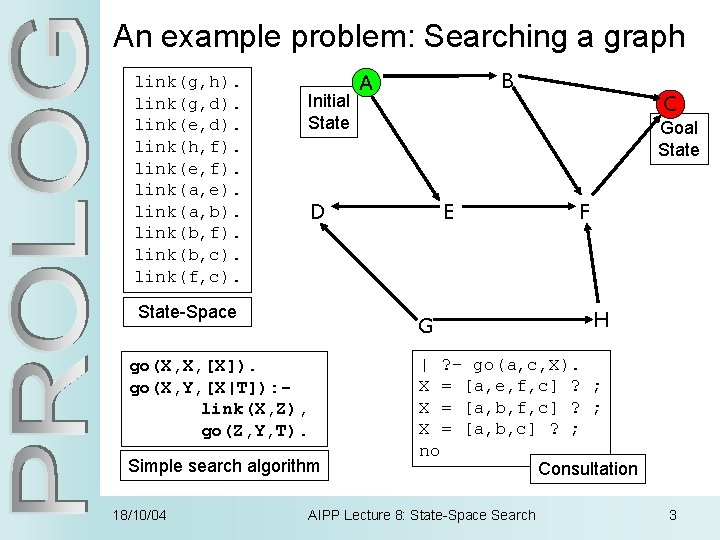State-Space Representation link(g, h). link(g, d). link(e, d). link(h, f). link(e, f). link(a, e). link(a, b). link(b, f). link(b, c). link(f, c). Initial state is the root A E D B F F C C C • An abstract representation of a state-space is a downwards growing tree. Connected nodes represent states in the domain. • The branching factor denotes how many new states you can move to from any state. This problem has an average of 2. • The depth of a node denotes how many moves away from the initial state it is. ‘C’ has two depths, 2 or 3. 18/10/04 AIPP Lecture 8: State-Space Search 4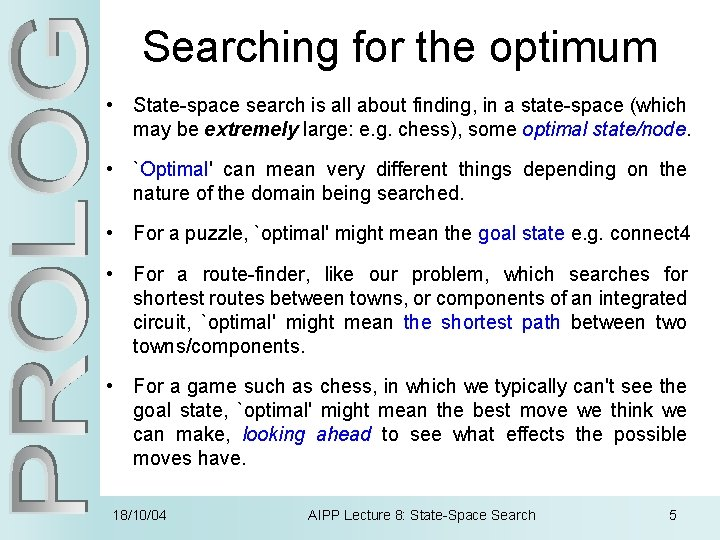Searching for the optimum • State-space search is all about finding, in a state-space (which may be extremely large: e. g. chess), some optimal state/node. • `Optimal' can mean very different things depending on the nature of the domain being searched. • For a puzzle, `optimal' might mean the goal state e. g. connect 4 • For a route-finder, like our problem, which searches for shortest routes between towns, or components of an integrated circuit, `optimal' might mean the shortest path between two towns/components. • For a game such as chess, in which we typically can't see the goal state, `optimal' might mean the best move we think we can make, looking ahead to see what effects the possible moves have. 18/10/04 AIPP Lecture 8: State-Space Search 5Implementing To implement state-space search in Prolog, we need: 1. A way of representing a state e. g. the board configuration 1. link(a, e). 2. A way of generating all of the next states reachable from a given state; • go(X, Y, [X|T]): - link(X, Z), go(Z, Y, T). 3. A way of determining whether a given state is the one we're looking for. Sometimes this might be the goal state (a finished puzzle, a completed route, a checkmate position); other times it might simply be the state we estimate is the best, using some evaluation function; • go(X, X, [X]). 4. A mechanism for controlling how we search the space. 18/10/04 AIPP Lecture 8: State-Space Search 6Depth-First Search A go(X, X, [X]). go(X, Y, [X|T]): link(X, Z), go(Z, Y, T). | ? - go(a, c, X). X = [a, e, f, c] ? ; X = [a, b, c] ? ; no E D B F F C C C • This simple search algorithm uses Prolog’s unification routine to find the first link from the current node then follows it. • It always follows the left-most branch of the search tree first; following it down until it either finds the goal state or hits a dead -end. It will then backtrack to find another branch to follow. = depth-first search. 18/10/04 AIPP Lecture 8: State-Space Search 7Iterative Deepening • If the optimal solution is the shortest path from the initial state to the goal state depth-first search will usually not find this. • We need to vary the depth at which we look for a solution; increasing the depth every time we have exhausted all nodes at a particular depth. • We can take advantage of Prolog’s backtracking to implement this very simply. Depth-First Iterative Deepening go(X, X, [X]). Check if current node is goal. go(X, Y, [X|T]): link(X, Z), go(Z, Y, T). go(X, Y, [Y|T]): go(X, Z, T), link(Z, Y). Find an intermediate node. 18/10/04 Check whether intermediate links with goal. AIPP Lecture 8: State-Space Search 8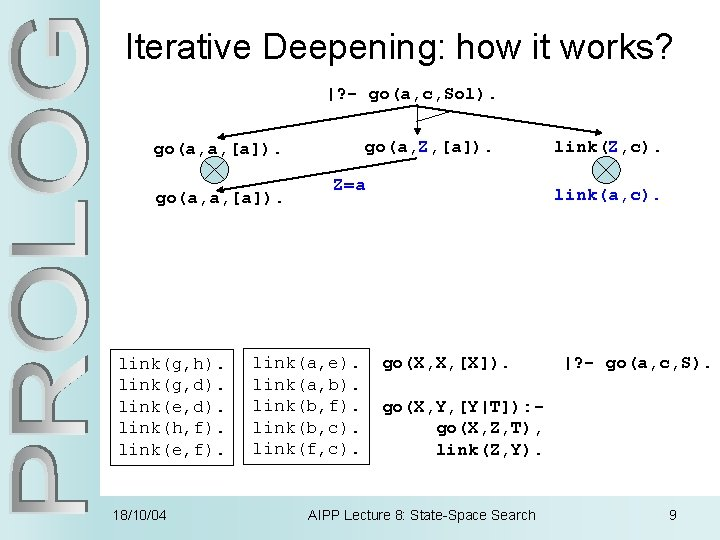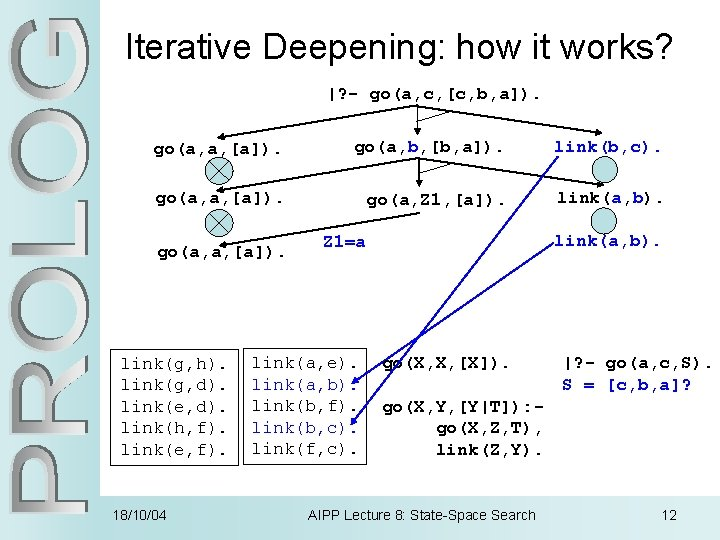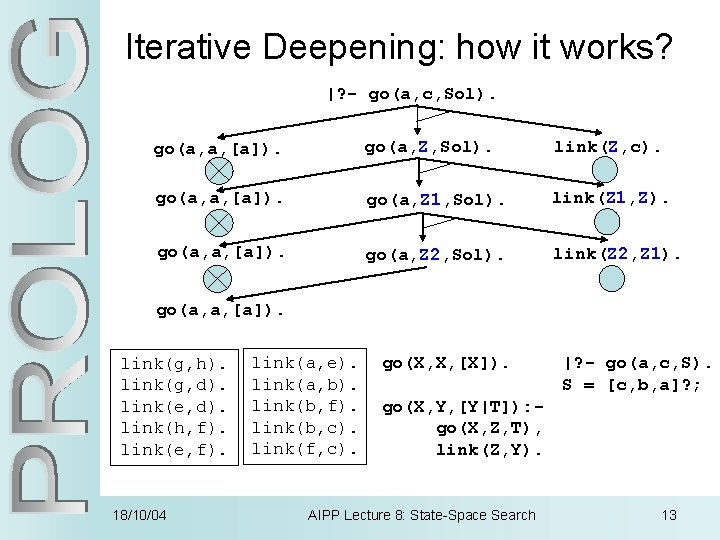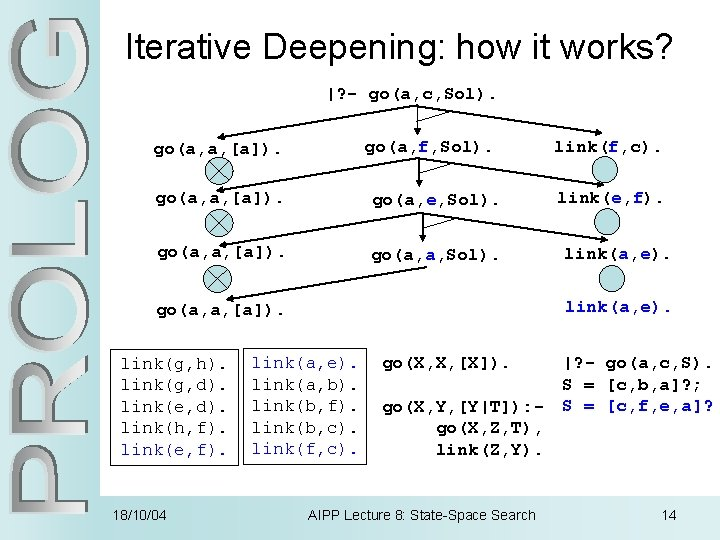Iterative Deepening (3) • Iterative Deepening search is quite useful as: – it is simple; – reaches a solution quickly, and – with minimal memory requirements as at any point in the search it is maintaining only one path back to the initial state. • However: – on each iteration it has to re-compute all previous levels and extend them to the new depth; – may not terminate (e. g. loop); – may not be able to handle complex state-spaces; – can’t be used in conjunction with problem-specific heuristics as keeps no memory of optional paths. 18/10/04 AIPP Lecture 8: State-Space Search 15Breadth-First Search A | ? - go(a, c, X). X = [a, b, c] ? ; X = [a, e, f, c] ? ; X = [a, b, f, c] ? ; no E Depth-first D = A, ED, FC, BFC, C Breadth-first = A, EB, DFFC, CC 1 st B F F C C C 2 nd 3 rd • A simple, common alternative to depth-first search is: breadth-first search. • This checks every node at one level of the space, before moving onto the next level. • It is distinct from iterative deepening as it maintains a list of alternative candidate nodes that can be expanded at each depth 18/10/04 AIPP Lecture 8: State-Space Search 16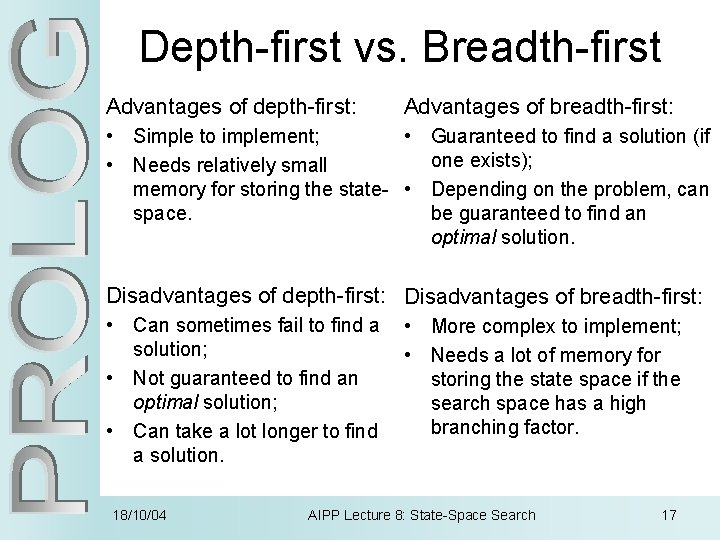Depth-first vs. Breadth-first Advantages of depth-first: Advantages of breadth-first: • Simple to implement; • Guaranteed to find a solution (if one exists); • Needs relatively small memory for storing the state- • Depending on the problem, can space. be guaranteed to find an optimal solution. Disadvantages of depth-first: Disadvantages of breadth-first: • Can sometimes fail to find a solution; • Not guaranteed to find an optimal solution; • Can take a lot longer to find a solution. 18/10/04 • More complex to implement; • Needs a lot of memory for storing the state space if the search space has a high branching factor. AIPP Lecture 8: State-Space Search 17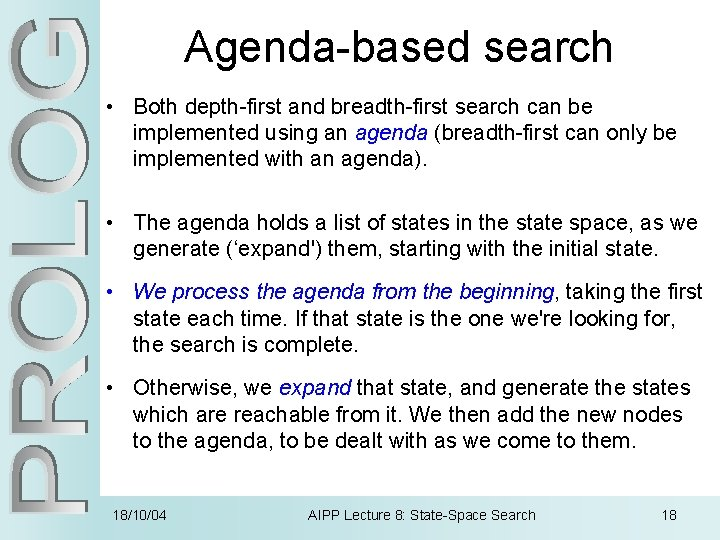Agenda-based search • Both depth-first and breadth-first search can be implemented using an agenda (breadth-first can only be implemented with an agenda). • The agenda holds a list of states in the state space, as we generate (‘expand') them, starting with the initial state. • We process the agenda from the beginning, taking the first state each time. If that state is the one we're looking for, the search is complete. • Otherwise, we expand that state, and generate the states which are reachable from it. We then add the new nodes to the agenda, to be dealt with as we come to them. 18/10/04 AIPP Lecture 8: State-Space Search 18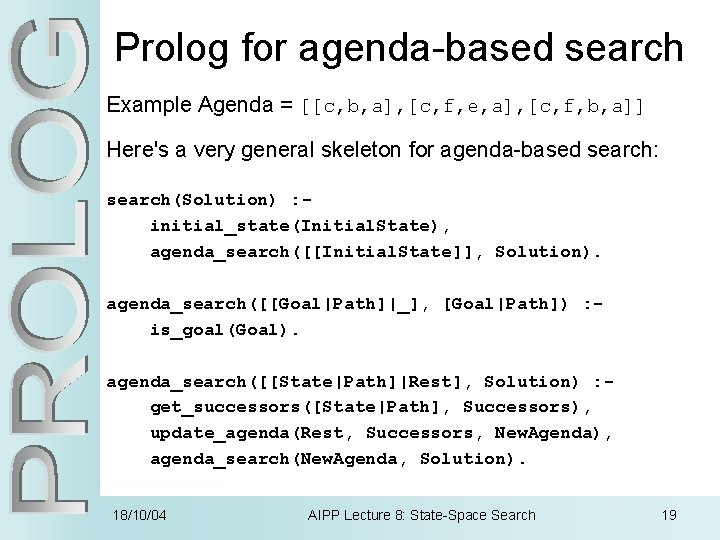Prolog for agenda-based search Example Agenda = [[c, b, a], [c, f, e, a], [c, f, b, a]] Here's a very general skeleton for agenda-based search: search(Solution) : initial_state(Initial. State), agenda_search([[Initial. State]], Solution). agenda_search([[Goal|Path]|_], [Goal|Path]) : is_goal(Goal). agenda_search([[State|Path]|Rest], Solution) : get_successors([State|Path], Successors), update_agenda(Rest, Successors, New. Agenda), agenda_search(New. Agenda, Solution). 18/10/04 AIPP Lecture 8: State-Space Search 19Prolog for agenda-based search (2) To complete the skeleton, we need to implement: • initial_state/1, – which creates the initial state for the state-space. • is_goal/1, – which succeeds if its argument is the goal state. • get_successors/2 – which generates all of the states which are reachable from a given state (should take advantage of findall/3, setof/3 or bagof/3 to achieve this). • update_agenda/2, – which adds new states to the agenda (usually using append/3). 18/10/04 AIPP Lecture 8: State-Space Search 20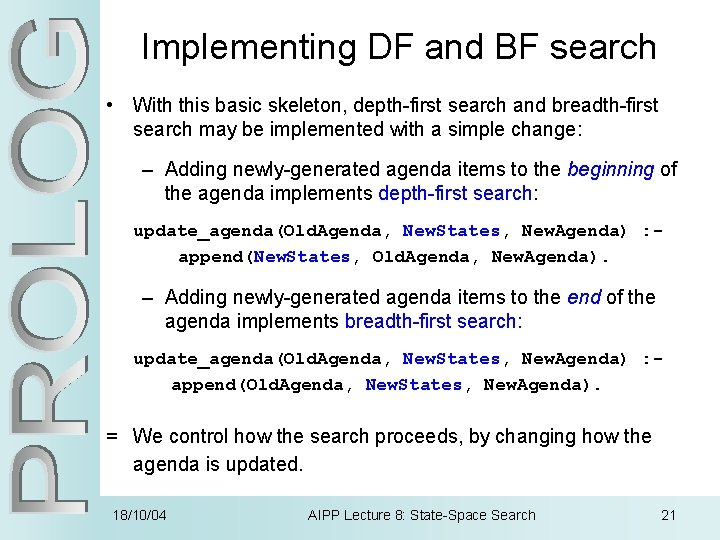Implementing DF and BF search • With this basic skeleton, depth-first search and breadth-first search may be implemented with a simple change: – Adding newly-generated agenda items to the beginning of the agenda implements depth-first search: update_agenda(Old. Agenda, New. States, New. Agenda) : append(New. States, Old. Agenda, New. Agenda). – Adding newly-generated agenda items to the end of the agenda implements breadth-first search: update_agenda(Old. Agenda, New. States, New. Agenda) : append(Old. Agenda, New. States, New. Agenda). = We control how the search proceeds, by changing how the agenda is updated. 18/10/04 AIPP Lecture 8: State-Space Search 21Summary • State-Space Search can be used to find optimal paths through problem spaces. • A state-space is represented as a downwards-growing tree with nodes representing states and branches as legal moves between states. • Prolog’s unification strategy allows a simplementation of depth-first search. • The efficiency of this can be improved by performing iterative deepening search (using backtracking). • Breadth-first search always finds the shortest path to the goal state. • Both depth and breadth-first search can be implemented using an agenda: – depth-first adds new nodes to the front of the agenda; – breadth-first adds new nodes to the end. 18/10/04 AIPP Lecture 8: State-Space Search 22